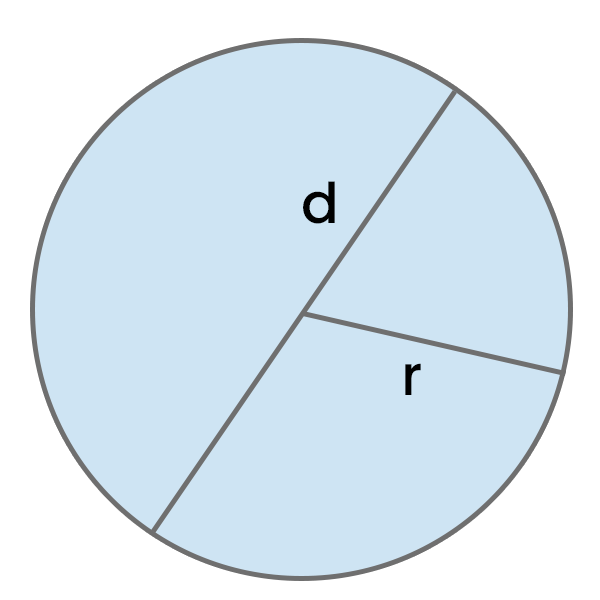# Area of a Circle Calculator

Calculate the area of a circle based on its radius (r) or diameter (d) in selected units, and see the detailed step-by-step calculation process.

Area = π * r²Result:

## How to use this calculator?

1. Enter the Circle Radius (r) or Circle Diameter (d) in the selected unit.
2. See the calculated Area of a Circle and if needed, change its unit.
3. See the detailed, step-by-step Calculation process.

## How to calculate Area of a Circle?

Multiply PI (approximately 3.14) by the squared Circle Radius.

Area of a Circle = π * r²
• π - Pi (~3,14)

If only the Diameter is given, divide it by 2 to obtain the Radius.

Example 1: Calculate the area of a circle with a radius of 8 cm.

Area = π * r²

Area = π * (8 cm)²

Area ≈ 3.14 * 64 cm²

Area ≈ 200.96 cm²

Example 2: Calculate the area of a circle with a diameter of 14 cm.

Radius = 14 cm / 2

Area = π * r²

Area = π * (7 cm)²

Area ≈ 3.14 * 49 cm²

Area ≈ 153.86 cm²